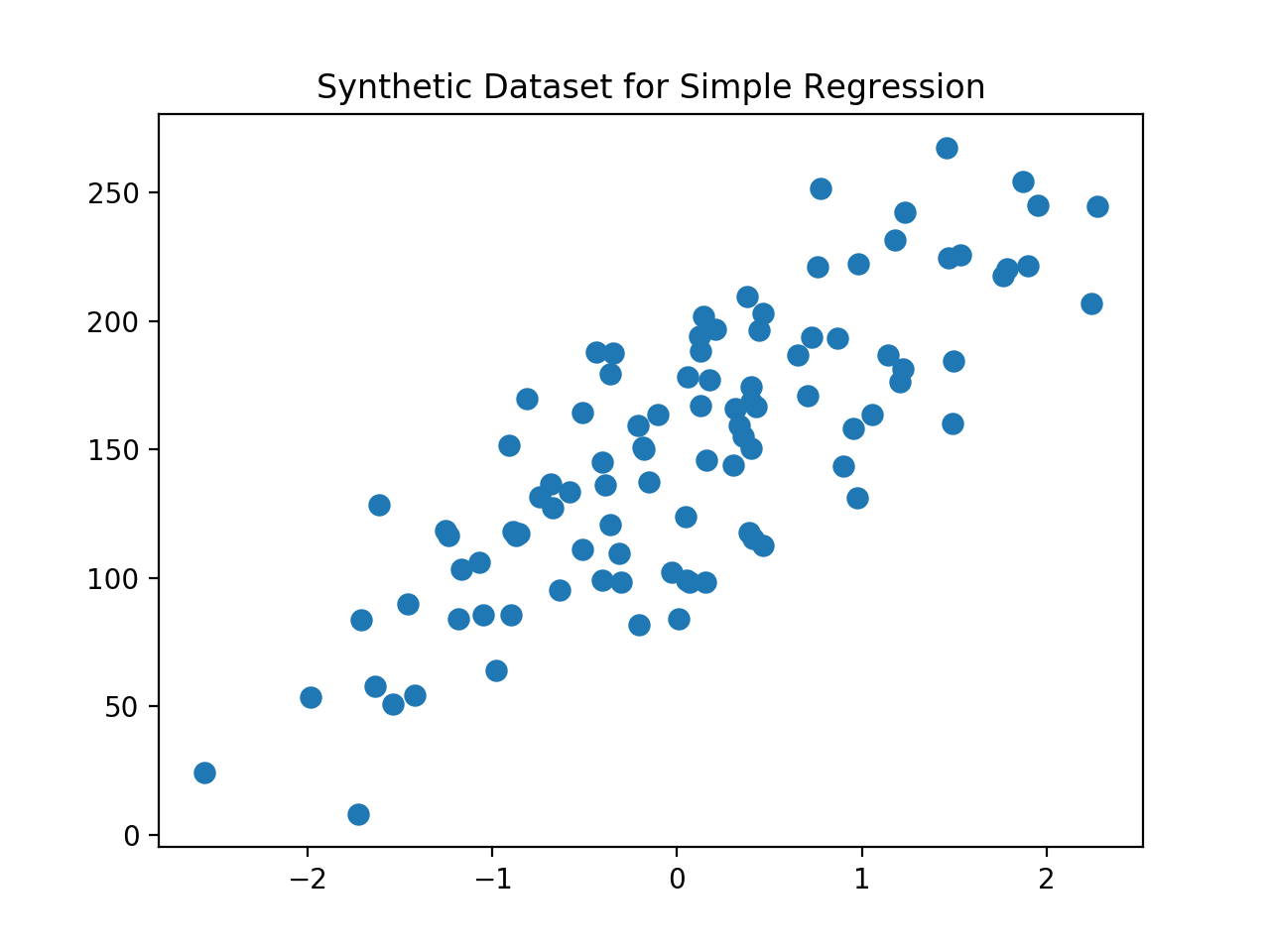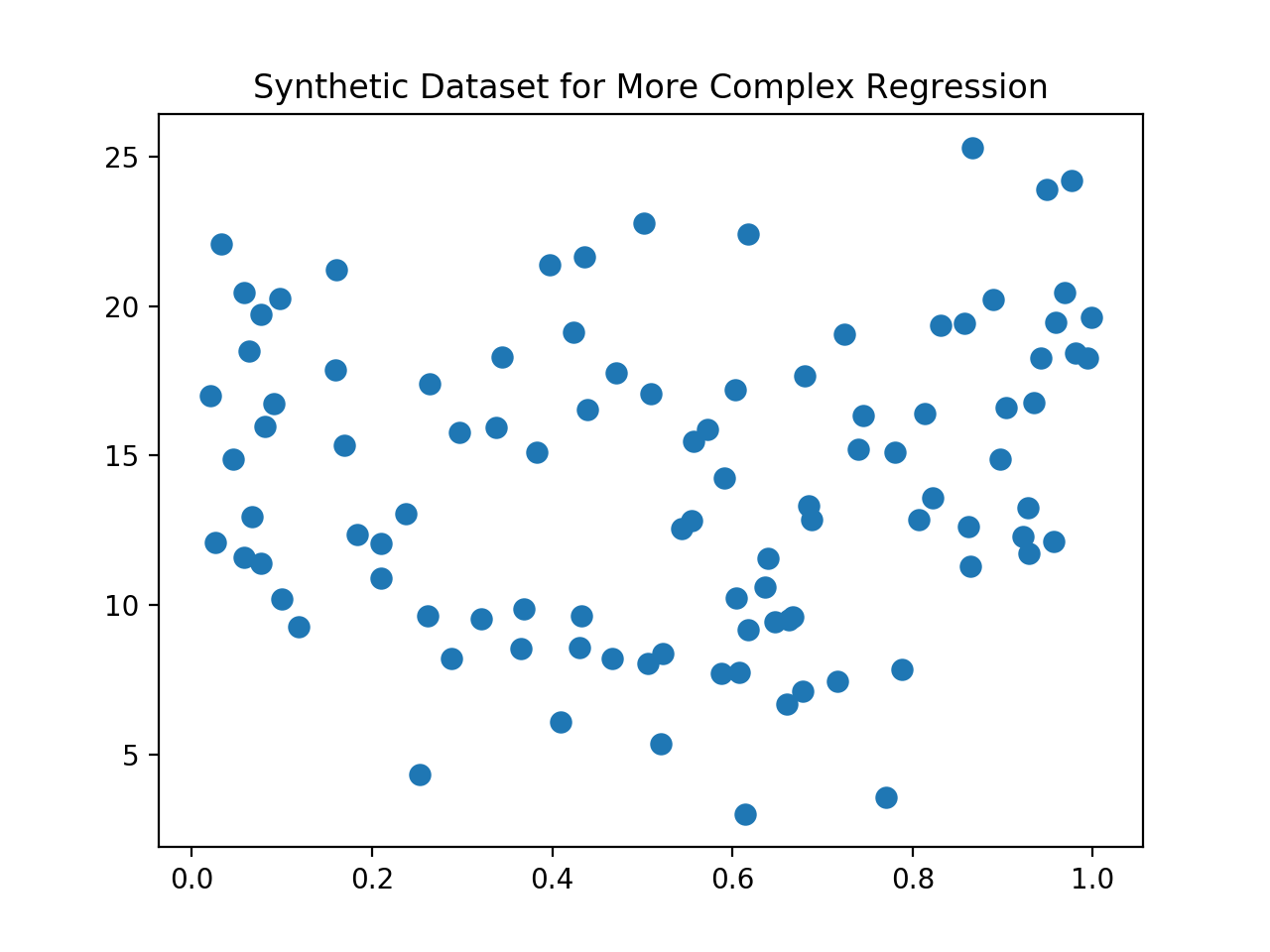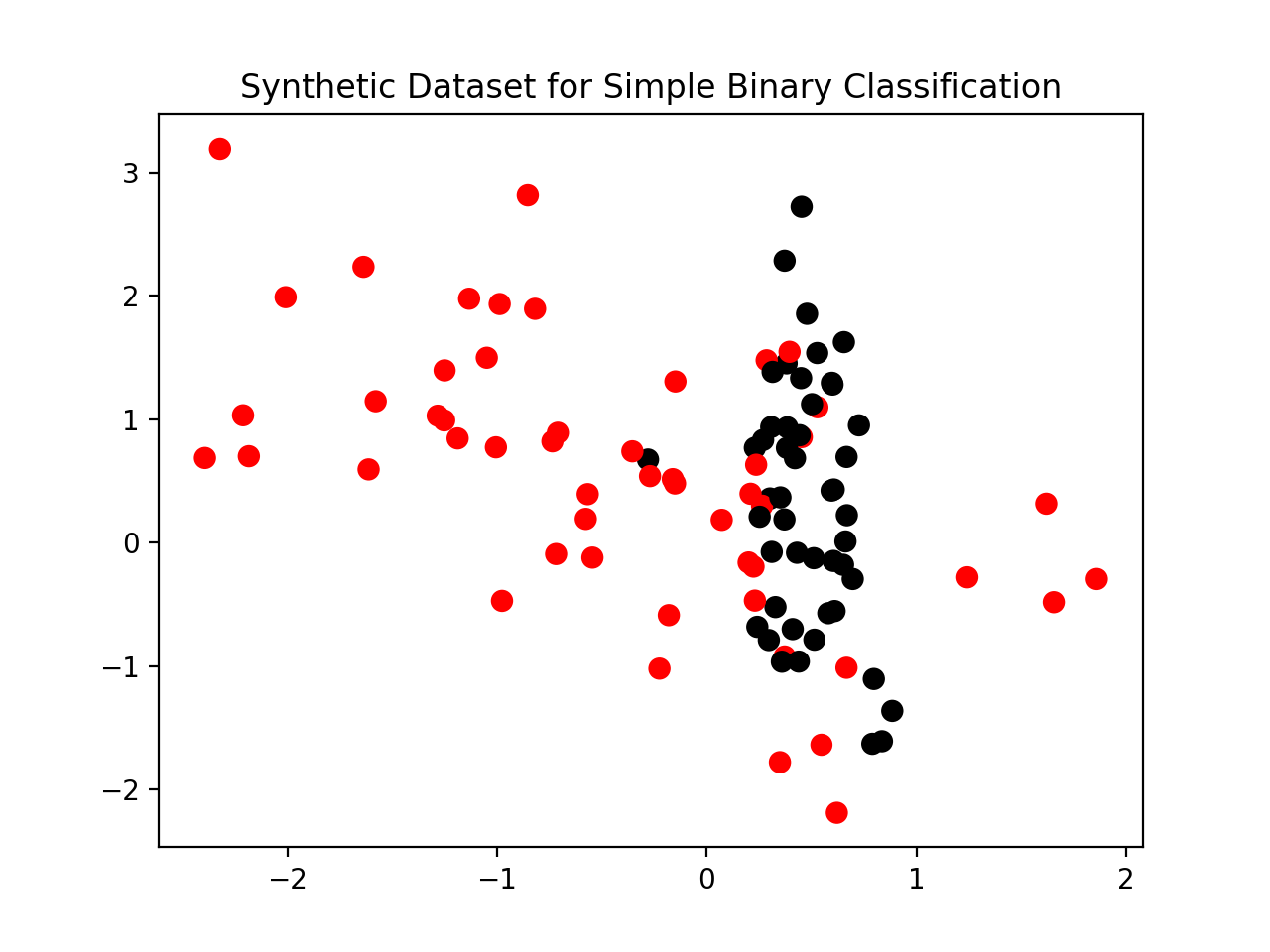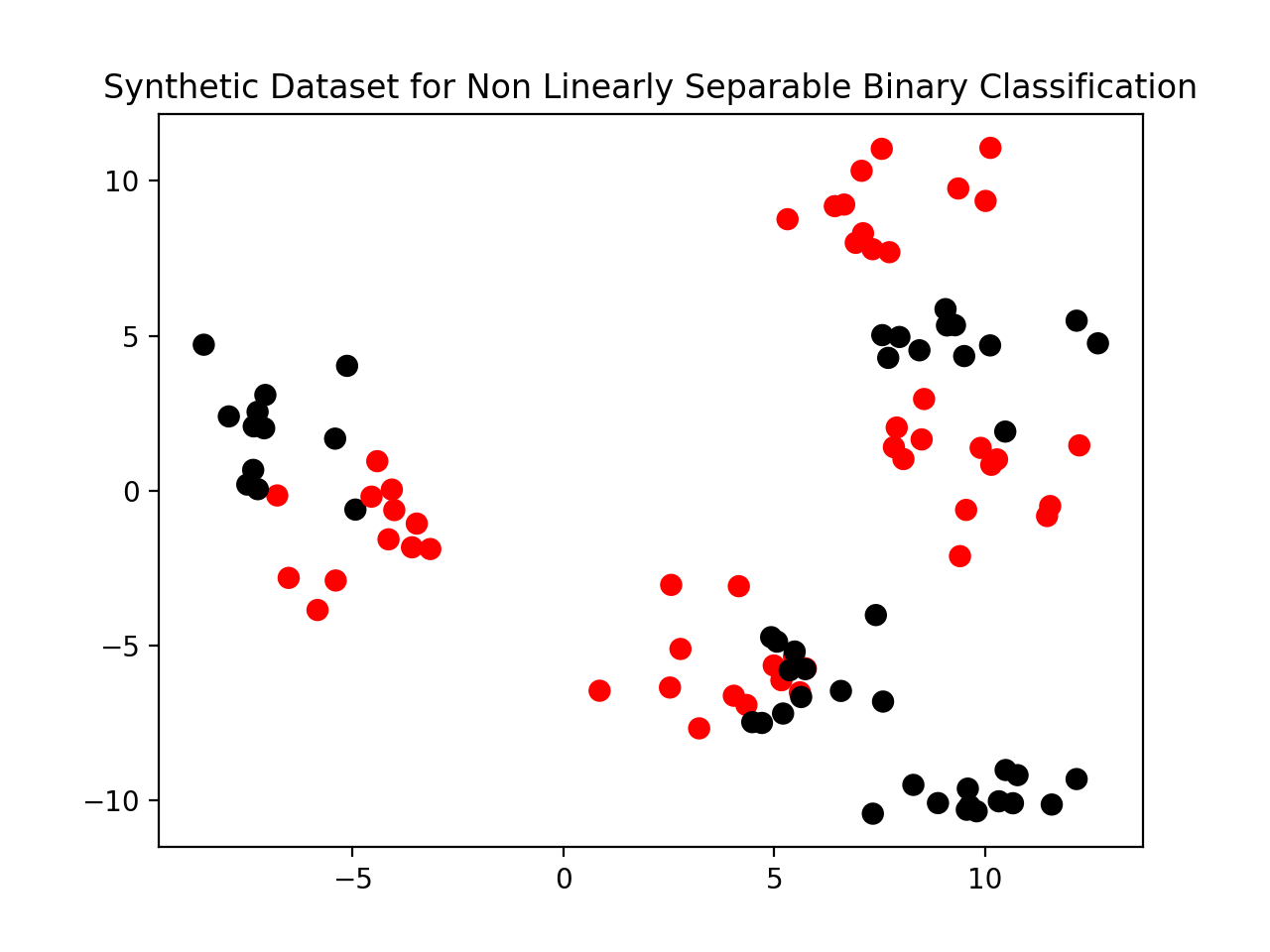# Synthetic Datasets

Scikit-Learn has a number of methods for generating synthetic datasets. Synthetic datasets are useful for explanatory purporses, because they can be made low dimensional. Low dimensional datasets only contain a small number of features, usally one or two. This makes them easy to explain and visualize.

Real world datasets, on the other hand, can often have a higher dimensional feature space. They may have dozens to hundreds, even thousands or millions, of features. So, some of the intuition gained from looking at low dimensional examples does not translate to high dimensional datasets.

Specifically, many high dimensional datasets have their data in corners, in some sense, with lots of empty space. This makes them difficult to visualize.

### Synthetic Dataset for Simple Regression

The following dataset has one informative input variable. The x-axis shows that feature’s value, and the y-axis shows the regression target.

%matplotlib notebook
import matplotlib.pyplot as plt
from sklearn.datasets import make_regression

plt.figure()
plt.title('Synthetic Dataset for Simple Regression')
X_R1, y_R1 = make_regression(n_samples = 100,
n_features=1,
n_informative=1,
bias = 150.0,
noise = 30,
random_state=0)
plt.scatter(X_R1,
y_R1,
marker= 'o',
s=50)

plt.show()

<IPython.core.display.Javascript object>### Synthetic Dataset for More Complex Regression

%matplotlib notebook
import matplotlib.pyplot as plt
from sklearn.datasets import make_friedman1

plt.figure()
plt.title('Synthetic Dataset for More Complex Regression')
X_F1, y_F1 = make_friedman1(n_samples = 100,
n_features = 7,
random_state=0)

plt.scatter(X_F1[:, 2],
y_F1,
marker= 'o',
s=50)
plt.show()

<IPython.core.display.Javascript object>### Synthetic Dataset for Simple Binary Classification

The following dataset has two classes with two informative features. The first feature is on the x-axis, and the second is on the y-axis. The color of each point shows which class that data instance is labeled.

In this case, these two classes are approximately linearly separable.

%matplotlib notebook
import matplotlib.pyplot as plt
from sklearn.datasets import make_classification
from matplotlib.colors import ListedColormap

cmap_bold = ListedColormap(['#FF0000',
'#00FF00',
'#0000FF',
'#000000'])

plt.figure()
plt.title('Synthetic Dataset for Simple Binary Classification')
X_C2, y_C2 = make_classification(n_samples = 100,
n_features=2,
n_redundant=0,
n_informative=2,
n_clusters_per_class=1,
flip_y = 0.1,
class_sep = 0.5,
random_state=0)
plt.scatter(X_C2[:, 0],
X_C2[:, 1],
c=y_C2,
marker= 'o',
s=50,
cmap=cmap_bold)

plt.show()

<IPython.core.display.Javascript object>### Synthetic Dataset for Complex Binary Classification

The data in the example below are not linearly separable.

%matplotlib notebook
import matplotlib.pyplot as plt
from sklearn.datasets import make_blobs
from matplotlib.colors import ListedColormap

cmap_bold = ListedColormap(['#FF0000',
'#00FF00',
'#0000FF',
'#000000'])

X_D2, y_D2 = make_blobs(n_samples = 100,
n_features = 2,
centers = 8,
cluster_std = 1.3,
random_state = 4)
y_D2 = y_D2 % 2
plt.figure()
plt.title('Synthetic Dataset for ' +
'Non Linearly Separable Binary Classification')
plt.scatter(X_D2[:,0],
X_D2[:,1],
c=y_D2,
marker= 'o',
s=50,
cmap=cmap_bold)
plt.show()

<IPython.core.display.Javascript object>